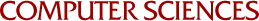## Statistical Properties of the Buddy System

Paul W. Purdom, Stephen M. Stigler
1969

The utilization of space and the running speed of the budy system are considered. Equations are derived that give various statistical properties of the buddy system. The bottom level with Poisson requests and exponential service times is investigated in great detail. Some equations are also given for investigating all levels at the same time and for non-Poisson requests. For the bottom level with Poisson requests and exponential service times the expected amount of space wasted by pairing full cells with empty cells and the amount of time spent by the bottom level requesting space from the next level is calculated for many rates of request. Asymptotic formulas are found which give a good fit to the calculated values. The results of a number of simulations of the buddy system are also given. These simulations indicate the behavior of many characteristics of the buddy system which can not be calculated in reasonable time with the formulas presented.